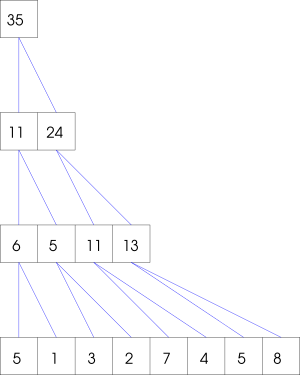Computing sum

Given is an array of n integers, such that n is power of two. The task is to compute the sum of all elements.

The simplest sequential way is as follows:

``````input int A[_];
output int sum = 0;

for (int i = 0; i < A.size; i++)
sum += A[i];
``````
Note that the time and work are equal, and linear in n. We can do better using parallelism:
``````input int A[_];
int n = A.size;
int Y[n];

output int sum;

pardo(i : n) Y[i] = A[i];

for (int t = 0; 2 ^ t < n; t++)
pardo(i : n / 2 ^ (t + 1))
Y[i] = Y[2 * i] + Y[2 * i + 1];

sum = Y;
``````
The program first copies the input array `A` to an auxiliary array `Y`. Then it proceeds in log n iterations; in each iteration the pairs of consecutive elements are added in parallel as follows:Hence, the work is still linear, but the time is only logarithmic.